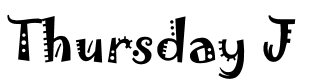## Thursday Night PoolSchedule## ***Home Team is listed LAST***Thursday H

 JANESVILLE BILLIARD LEAGUE Thursday H Bar Captain PHONE 1 ALTA MAY'S PLACE 1 2 HANSON'S HOLLOW BODIE TRACY 608-921-1889 3 RILEY'S 1 KEVIN RILEY 608-359-3216 4 SHADY NOOK 3 TROY JANSSEN 608-290-3785 5 SNEAKERS 2 TIM GREEN 608-751-7815 6 FRIENDLY TAP BRIAN JOHNSON 608-921-0347 7 RILEY'S 2 RICH HILLER 608-359-2490 8 SHADY NOOK 2 WOODY 815-307-1026 ***Home team listed last Week  1 Week  2 Week  3 Week  4 Week  5 Week  6 Week  7 10/5/23 10/12/23 10/19/23 10/26/23 11/2/23 11/9/23 11/16/23 1 at  8 4 at  8 8 at  7 3 at  8 8 at  6 2 at  8 8 at  5 2 at  7 5 at  3 1 at  6 4 at  2 7 at  5 3 at  1 6 at  4 3 at  6 6 at  2 2 at  5 5 at  1 1 at  4 4 at  7 7 at  3 4 at  5 7 at  1 3 at  4 6 at  7 2 at  3 5 at  6 1 at  2 Week  8 Week  9 Week  10 Week  11 Week  12 Week  13 Week  14 11/30/23 12/7/23 12/14/23 12/21/23 1/4/24 1/11/24 1/18/24 8 at  1 8 at  4 7 at  8 8 at  3 6 at  8 8 at  2 5 at  8 7 at  2 3 at  5 6 at  1 2 at  4 5 at  7 1 at  3 4 at  6 6 at  3 2 at  6 5 at  2 1 at  5 4 at  1 7 at  4 3 at  7 5 at  4 1 at  7 4 at  3 7 at  6 3 at  2 6 at  5 2 at  1 Week  15 Week  16 Week  17 Week  18 Week  19 Week  20 Week  21 1/25/24 2/1/24 2/8/24 2/15/24 2/22/24 2/29/24 3/7/24 1 at  8 4 at  8 8 at  7 3 at  8 8 at  6 2 at  8 8 at  5 2 at  7 5 at  3 1 at  6 4 at  2 7 at  5 3 at  1 6 at  4 3 at  6 6 at  2 2 at  5 5 at  1 1 at  4 4 at  7 7 at  3 4 at  5 7 at  1 3 at  4 6 at  7 2 at  3 5 at  6 1 at  2JANESVILLE BILLIARD LEAGUE THURSDAY I Bar Captain 1 SNEAKERS 1 PHILIP FJALSTAD 608-751-9159 2 SHADY NOOK 1 JEN SPEER 608-289-2875 3 WILLOWDALE 1 GREG BRUNHOEFER 608-359-2807 4 THE TABLE CHRIS STEPHENSON 608-436-2670 5 RIDERS IN 1 ASSHOLE 608-247-4779 6 BOGEYS JOSH PEARSON 608-290-8432 ***Home team listed last Week  1 Week  2 Week  3 Week  4 Week  5 10/5/2023 10/12/2023 10/19/2023 10/26/2023 11/2/2023 1 at  6 3 at  6 6 at  5 2 at  6 6 at  4 2 at  5 4 at  2 1 at  4 3 at  1 5 at  3 3 at  4 5 at  1 2 at  3 4 at  5 1 at  2 Week  6 Week  7 Week  8 Week  9 Week  10 11/9/2023 11/16/2023 11/30/2023 12/7/2023 12/14/2023 6 at  1 6 at  3 5 at  6 6 at  2 4 at  6 5 at  2 2 at  4 4 at  1 1 at  3 3 at  5 4 at  3 1 at  5 3 at  2 5 at  4 2 at  1 Week  11 Week  12 Week  13 Week  14 Week  15 12/21/2023 1/4/2024 1/11/2024 1/18/2024 1/25/2024 1 at  6 3 at  6 6 at  5 2 at  6 6 at  4 2 at  5 4 at  2 1 at  4 3 at  1 5 at  3 3 at  4 5 at  1 2 at  3 4 at  5 1 at  2 Week  16 Week  17 Week  18 Week  19 Week  20 2/1/2024 2/8/2024 2/15/2024 2/22/2024 2/29/2024 6 at  1 6 at  3 5 at  6 6 at  2 4 at  6 5 at  2 2 at  4 4 at  1 1 at  3 3 at  5 4 at  3 1 at  5 3 at  2 5 at  4 2 at  1JANESVILLE BILLIARD LEAGUE THURSDAY J Bar Captain 1 WIGGY'S JIM JENSEN 608-752-3953 2 HAMMY'S 1 BRUCE HAMILTON 608-289-4087 3 BAD BRAD'S MIKE DAVIS 608-751-6867 4 ALTA MAY'S PLACE 1 BUDHA 608-449-3546 5 HAMMY'S 2 STEVE MAHLUM 608-449-8626 6 WILLOWDALE 2 JOSH KELM 608-201-5138 ***Home team listed last Week  1 Week  2 Week  3 Week  4 Week  5 10/5/2023 10/12/2023 10/19/2023 10/26/2023 11/2/2023 1 at  6 3 at  6 6 at  5 2 at  6 6 at  4 2 at  5 4 at  2 1 at  4 3 at  1 5 at  3 3 at  4 5 at  1 2 at  3 4 at  5 1 at  2 Week  6 Week  7 Week  8 Week  9 Week  10 11/9/2023 11/16/2023 11/30/2023 12/7/2023 12/14/2023 6 at  1 6 at  3 5 at  6 6 at  2 4 at  6 5 at  2 2 at  4 4 at  1 1 at  3 3 at  5 4 at  3 1 at  5 3 at  2 5 at  4 2 at  1 Week  11 Week  12 Week  13 Week  14 Week  15 12/21/2023 1/4/2024 1/11/2024 1/18/2024 1/25/2024 1 at  6 3 at  6 6 at  5 2 at  6 6 at  4 2 at  5 4 at  2 1 at  4 3 at  1 5 at  3 3 at  4 5 at  1 2 at  3 4 at  5 1 at  2 Week  16 Week  17 Week  18 Week  19 Week  20 2/1/2024 2/8/2024 2/15/2024 2/22/2024 2/29/2024 6 at  1 6 at  3 5 at  6 6 at  2 4 at  6 5 at  2 2 at  4 4 at  1 1 at  3 3 at  5 4 at  3 1 at  5 3 at  2 5 at  4 2 at  1

Thursday K